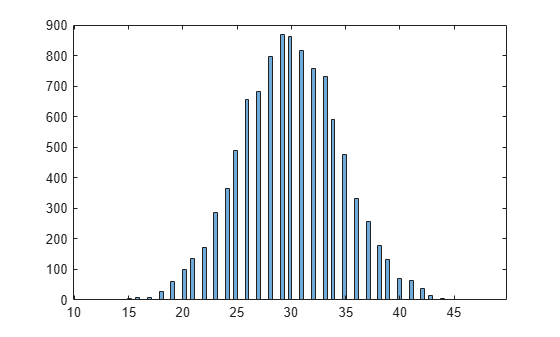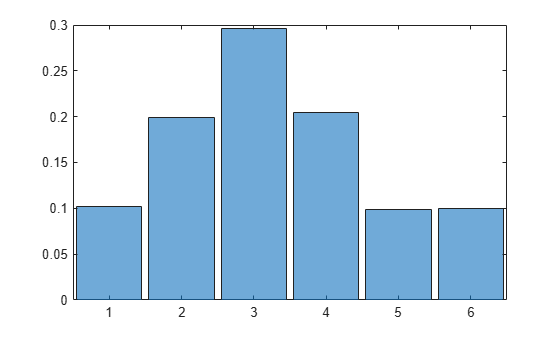## Generating Pseudorandom Numbers

Pseudorandom numbers are generated by deterministic algorithms. They are "random" in the sense that, on average, they pass statistical tests regarding their distribution and correlation. They differ from true random numbers in that they are generated by an algorithm, rather than a truly random process.

Random number generators (RNGs) like those in MATLAB® are algorithms for generating pseudorandom numbers with a specified distribution.

For more information on the GUI for generating random numbers from supported distributions, see Explore the Random Number Generation UI.

### Common Pseudorandom Number Generation Methods

Methods for generating pseudorandom numbers usually start with uniform random numbers, like the MATLAB `rand` function produces. The methods described in this section detail how to produce random numbers from other distributions.

#### Direct Methods

Direct methods directly use the definition of the distribution.

For example, consider binomial random numbers. A binomial random number is the number of heads in $N$ tosses of a coin with probability $p$ of a heads on any single toss. If you generate $N$ uniform random numbers on the interval `(0,1)` and count the number less than $p$, then the count is a binomial random number with parameters $N$ and $p$.

This function is a simple implementation of a binomial RNG using the direct approach:

```function X = directbinornd(N,p,m,n) X = zeros(m,n); % Preallocate memory for i = 1:m*n u = rand(N,1); X(i) = sum(u < p); end end ```

For example:

```rng('default') % For reproducibility X = directbinornd(100,0.3,1e4,1); histogram(X,101)```The `binornd` function uses a modified direct method, based on the definition of a binomial random variable as the sum of Bernoulli random variables.

You can easily convert the previous method to a random number generator for the Poisson distribution with parameter $\lambda$. The Poisson Distribution is the limiting case of the binomial distribution as $N$ approaches infinity, $p$ approaches zero, and $Np$ is held fixed at $\lambda$. To generate Poisson random numbers, create a version of the previous generator that inputs $\lambda$ rather than $N$ and $p$, and internally sets $N$ to some large number and $p$ to $\lambda /N$.

The `poissrnd` function actually uses two direct methods:

• A waiting time method for small values of $\lambda$

• A method due to Ahrens and Dieter for larger values of $\lambda$

#### Inversion Methods

Inversion methods are based on the observation that continuous cumulative distribution functions (cdfs) range uniformly over the interval `(0,1)`. If $u$ is a uniform random number on `(0,1)`, then using $X={F}^{-1}\left(U\right)$ generates a random number $X$ from a continuous distribution with specified cdf $F$.

For example, the following code generates random numbers from a specific Exponential Distribution using the inverse cdf and the MATLAB® uniform random number generator `rand`:

```rng('default') % For reproducibility mu = 1; X = expinv(rand(1e4,1),mu);```

Compare the distribution of the generated random numbers to the pdf of the specified exponential.

```numbins = 50; h = histogram(X,numbins,'Normalization','pdf'); hold on x = linspace(h.BinEdges(1),h.BinEdges(end)); y = exppdf(x,mu); plot(x,y,'LineWidth',2) hold off```Inversion methods also work for discrete distributions. To generate a random number $X$ from a discrete distribution with probability mass vector $P\left(X={x}_{i}\right)={p}_{i}$ where ${x}_{0}<{x}_{1}<{x}_{2}<...$, generate a uniform random number $u$ on `(0,1)` and then set $X={x}_{i}$ if $F\left({x}_{i-1}\right).

For example, the following function implements an inversion method for a discrete distribution with probability mass vector $p$:

```function X = discreteinvrnd(p,m,n) X = zeros(m,n); % Preallocate memory for i = 1:m*n u = rand; I = find(u < cumsum(p)); X(i) = min(I); end end ```

Use the function to generate random numbers from any discrete distribution.

```p = [0.1 0.2 0.3 0.2 0.1 0.1]; % Probability mass function (pmf) values X = discreteinvrnd(p,1e4,1);```

Alternatively, you can use the `discretize` function to generate discrete random numbers.

```X = discretize(rand(1e4,1),[0 cusmsum(p)]); ```

Plot the histogram of the generated random numbers, and confirm then the distribution follows the specified pmf values.

`histogram(categorical(X),'Normalization','probability')`#### Acceptance-Rejection Methods

The functional form of some distributions makes it difficult or time-consuming to generate random numbers using direct or inversion methods. Acceptance-rejection methods provide an alternative in these cases.

Acceptance-rejection methods begin with uniform random numbers, but require an additional random number generator. If your goal is to generate a random number from a continuous distribution with pdf $f$, acceptance-rejection methods first generate a random number from a continuous distribution with pdf $g$ satisfying $f\left(x\right)\le cg\left(x\right)$ for some $c$ and all $x$.A continuous acceptance-rejection RNG proceeds as follows:

1. Chooses a density $g$.

2. Finds a constant $c$ such that $f\left(x\right)/g\left(x\right)\le c$ for all $x$.

3. Generates a uniform random number $u$.

4. Generates a random number $v$ from $g$.

5. If $cu\le f\left(v\right)/g\left(v\right)$, accepts and returns $v$. Otherwise, rejects $v$ and goes to step 3.

For efficiency, a "cheap" method is necessary for generating random numbers from $g$, and the scalar $c$ should be small. The expected number of iterations to produce a single random number is $c$.

The following function implements an acceptance-rejection method for generating random numbers from pdf $f$ given $f$, $g$, the RNG `grnd` for $g$, and the constant $c$:

```function X = accrejrnd(f,g,grnd,c,m,n) X = zeros(m,n); % Preallocate memory for i = 1:m*n accept = false; while accept == false u = rand(); v = grnd(); if c*u <= f(v)/g(v) X(i) = v; accept = true; end end end end ```

For example, the function $f\left(x\right)=x{e}^{-{x}^{2}/2}$ satisfies the conditions for a pdf on $\left[0,\infty \right)$ (nonnegative and integrates to 1). The exponential pdf with mean 1, $f\left(x\right)={e}^{-x}$, dominates $g$ for $c$ greater than about 2.2. Thus, you can use `rand` and `exprnd` to generate random numbers from $f$:

```f = @(x)x.*exp(-(x.^2)/2); g = @(x)exp(-x); grnd = @()exprnd(1); rng('default') % For reproducibility X = accrejrnd(f,g,grnd,2.2,1e4,1);```

The pdf $f$ is actually a Rayleigh Distribution with shape parameter 1. This example compares the distribution of random numbers generated by the acceptance-rejection method with those generated by `raylrnd`:

```Y = raylrnd(1,1e4,1); histogram(X) hold on histogram(Y) legend('A-R RNG','Rayleigh RNG')```The `raylrnd` function uses a transformation method, expressing a Rayleigh random variable in terms of a chi-square random variable, which you compute using `randn`.

Acceptance-rejection methods also work for discrete distributions. In this case, the goal is to generate random numbers from a distribution with probability mass ${P}_{p}\left(X=i\right)={p}_{i}$, assuming that you have a method for generating random numbers from a distribution with probability mass ${P}_{q}\left(X=i\right)={q}_{i}$. The RNG proceeds as follows:

1. Chooses a density ${P}_{q}$.

2. Finds a constant $c$ such that ${p}_{i}/{q}_{i}\le c$ for all $i$.

3. Generates a uniform random number $u$.

4. Generates a random number $v$ from ${P}_{q}$.

5. If $cu\le {p}_{v}/{q}_{v}$, accepts and returns $v$. Otherwise, rejects $v$ and goes to step 3.